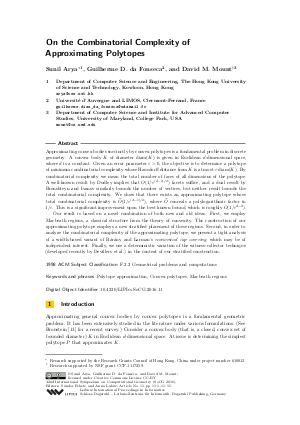Document# On the Combinatorial Complexity of Approximating Polytopes

### Authors Sunil Arya, Guilherme D. da Fonseca, David M. Mount## File

LIPIcs.SoCG.2016.11.pdf
• Filesize: 0.68 MB
• 15 pages

## Cite As

Sunil Arya, Guilherme D. da Fonseca, and David M. Mount. On the Combinatorial Complexity of Approximating Polytopes. In 32nd International Symposium on Computational Geometry (SoCG 2016). Leibniz International Proceedings in Informatics (LIPIcs), Volume 51, pp. 11:1-11:15, Schloss Dagstuhl - Leibniz-Zentrum für Informatik (2016)
https://doi.org/10.4230/LIPIcs.SoCG.2016.11

## Abstract

Approximating convex bodies succinctly by convex polytopes is a fundamental problem in discrete geometry. A convex body K of diameter \$diam(K)\$ is given in Euclidean d-dimensional space, where \$d\$ is a constant. Given an error parameter eps > 0, the objective is to determine a polytope of minimum combinatorial complexity whose Hausdorff distance from K is at most eps diam(K). By combinatorial complexity we mean the total number of faces of all dimensions of the polytope. A well-known result by Dudley implies that O(1/eps^{(d-1)/2}) facets suffice, and a dual result by Bronshteyn and Ivanov similarly bounds the number of vertices, but neither result bounds the total combinatorial complexity. We show that there exists an approximating polytope whose total combinatorial complexity is O-tilde(1/eps^{(d-1)/2}), where O-tilde conceals a polylogarithmic factor in 1/eps. This is an improvement upon the best known bound, which is roughly O(1/eps^{d-2}). Our result is based on a novel combination of both new and old ideas. First, we employ Macbeath regions, a classical structure from the theory of convexity. The construction of our approximating polytope employs a new stratified placement of these regions. Second, in order to analyze the combinatorial complexity of the approximating polytope, we present a tight analysis of a width-based variant of Barany and Larman's economical cap covering, which may be of independent interest. Finally, we use a deterministic variation of the witness-collector technique (developed recently by Devillers et al.) in the context of our stratified construction.
##### Keywords
• Polytope approximation
• Convex polytopes
• Macbeath regions

## Metrics

• Access Statistics
• Total Accesses (updated on a weekly basis)
0

## References

1. P. K. Agarwal, S. Har-Peled, and K. R. Varadarajan. Approximating extent measures of points. J. Assoc. Comput. Mach., 51:606-635, 2004.2. G. E. Andrews. A lower bound for the volumes of strictly convex bodies with many boundary points. Trans. Amer. Math. Soc., 106:270-279, 1963.3. S. Arya, G. D. da Fonseca, and D. M. Mount. Optimal area-sensitive bounds for polytope approximation. In Proc. 28th Annu. Sympos. Comput. Geom., pages 363-372, 2012.4. S. Arya, T. Malamatos, and D. M. Mount. The effect of corners on the complexity of approximate range searching. Discrete Comput. Geom., 41:398-443, 2009.5. S. Arya, D. M. Mount, and J. Xia. Tight lower bounds for halfspace range searching. Discrete Comput. Geom., 47:711-730, 2012. URL: http://dx.doi.org/10.1007/s00454-012-9412-x.
6. I. Bárány. Intrinsic volumes and f-vectors of random polytopes. Math. Ann., 285:671-699, 1989.7. I. Bárány. The technique of M-regions and cap-coverings: A survey. Rend. Circ. Mat. Palermo, 65:21-38, 2000.8. I. Bárány. Extremal problems for convex lattice polytopes: A survey. Contemp. Math., 453:87-103, 2008.9. I. Bárány and D. G. Larman. Convex bodies, economic cap coverings, random polytopes. Mathematika, 35:274-291, 1988.10. K. Böröczky, Jr. Approximation of general smooth convex bodies. Adv. Math., 153:325-341, 2000.11. H. Brönnimann, B. Chazelle, and J. Pach. How hard is halfspace range searching. Discrete Comput. Geom., 10:143-155, 1993.12. E. M. Bronshteyn and L. D. Ivanov. The approximation of convex sets by polyhedra. Siberian Math. J., 16:852-853, 1976.13. E. M. Bronstein. Approximation of convex sets by polytopes. J. Math. Sci., 153(6):727-762, 2008.14. K. L. Clarkson. Building triangulations using ε-nets. In Proc. 38th Annu. ACM Sympos. Theory Comput., pages 326-335, 2006.15. O. Devillers, M. Glisse, and X. Goaoc. Complexity analysis of random geometric structures made simpler. In Proc. 29th Annu. Sympos. Comput. Geom., pages 167-176, 2013.16. R. M. Dudley. Metric entropy of some classes of sets with differentiable boundaries. J. Approx. Theory, 10(3):227-236, 1974.17. G. Ewald, D. G. Larman, and C. A. Rogers. The directions of the line segments and of the r-dimensional balls on the boundary of a convex body in Euclidean space. Mathematika, 17:1-20, 1970.18. P. M. Gruber. Asymptotic estimates for best and stepwise approximation of convex bodies I. Forum Math., 5:521-537, 1993.19. S. Har-Peled. Geometric approximation algorithms. Number 173 in Mathematical surveys and monographs. American Mathematical Society, 2011.20. F. John. Extremum problems with inequalities as subsidiary conditions. In Studies and Essays Presented to R. Courant on his 60th Birthday, pages 187-204. Interscience Publishers, Inc., New York, 1948.21. A. M. Macbeath. A theorem on non-homogeneous lattices. Ann. of Math., 56:269-293, 1952.22. P. McMullen. The maximum numbers of faces of a convex polytope. Mathematika, 17:179-184, 1970.23. R. Schneider. Polyhedral approximation of smooth convex bodies. J. Math. Anal. Appl., 128:470-474, 1987.X

Feedback for Dagstuhl Publishing